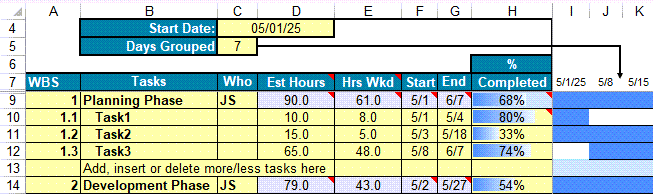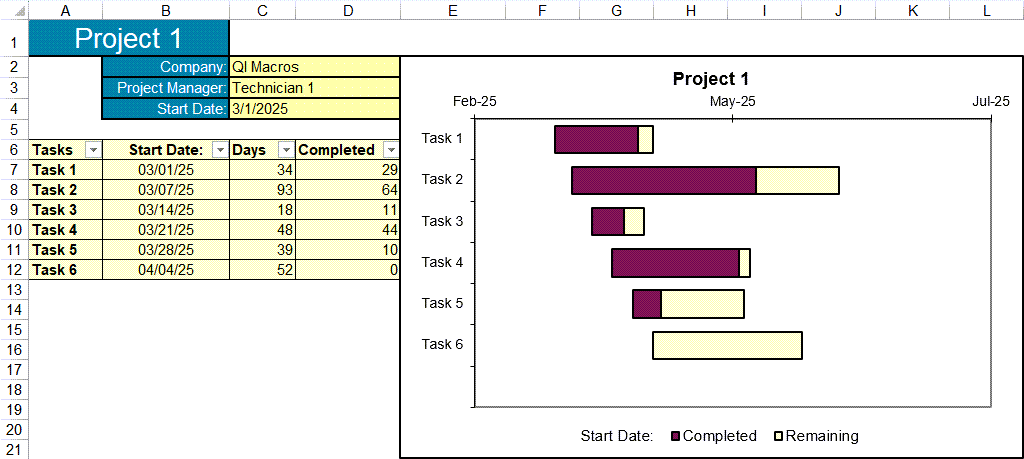# Looking for a Gantt Chart Template in Excel?

What is a Gantt Chart? Gantt charts are a valuable project planning tool that visually show the timing and length of project tasks using bars.

### QI Macros Gantt chart template is compatible with both PC and Mac.### How to create a Gantt chart using QI Macros template1. Input the start date in cell C4.
2. Input the "Days Grouped" in C5. Days grouped defines the # of days represented by each column.
3. Identify major project phases.
4. Identify the tasks under each phase.
5. For each task, input the number of hours required and the start and end dates.
You can type values into the cells or save time by using some of the templates built in formulas.
• Task 1: Input the start date. There are two ways to input end dates:
• Update the existing formula in the cell which equals the start date plus a # of days (i.e. E12 + 3)
• Type a date over the formula
• Subsequent Tasks: Start date is set to equal the end date for the previous task. This formula can be overridden by inputting a date into the cell.
6. For each phase, the values are calculated from the tasks. These can be overridden if desired.
7. Input the % complete. The template will shade part of the cell to indicate the %.

#### To expand the Gantt Chart Template:

• Add tasks: select the row labeled "Add, insert, or delete more tasks he re" then insert a row. This will properly update the summary formulas for the phase.
• Add phases: just type into the cells below the example
• Add rows to the bottom of the table: select the last row, then select and drag down the black box that appears.### How to use QI Macros Percent Complete Gantt Chart TemplateJust input task descriptions, start dates and total days vs days completed. The percent completed bars are automatically drawn.